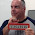## Tuesday, October 22, 2013

### Generating random numbers part IThere are times for statistical or testing reasons you need a random number. Computers have always struggled to generate truly random numbers, therefore, they use a process called psuedorandomness. Which is defined by Wikipedia as:

A pseudorandom process is a process that appears to be random but it is not. Pseudorandom sequences typically exhibit statistical randomness while being generated by an entirely deterministic causal process.

But how generate one on an IBM i (AS400)?

Fortunately there are two easy methods I have encountered and used:

1. CEERAN0 API (that is CEERAN-zero), which I will discuss here.
2. RAND SQL function, which I will describe in part II.

In my examples I am going to generate 1 million random numbers, and write them to a DDS file, RANDOM_F, which is shown below:

 ``` A R RANDOM_FR A KEY 7P 0 ```

The contents of this file will then be used by other programs for testing.

Below is an example of how I could use the CEERAN0 API.

 ```01 H dftactgrp(*no) 02 FRANDOM_F O E DISK 03 D CEERAN0 PR 04 D 10I 0 05 D 8F 06 D 12A options(*omit) 07 D LowNbr S like(KEY) 08 D HighNbr S like(KEY) 09 D Range S like(KEY) 11 D i S 10U 0 12 D RandomNbr S 8F 13 D Seed S 10I 0 /free 14 HighNbr = 1000000 ; 15 LowNbr = 0 ; 16 Range = (HighNbr - LowNbr) + 1 ; 17 for i = 1 to 1000000 ; 18 CEERAN0(Seed:RandomNbr:*omit) ; 19 KEY = %int(RandomNbr * Range) ; 20 write RANDOM_FR ; 21 endfor; 22 *inlr = *on ; ```

I have coded the API as a procedure on line 3. It has three parameters:

• Seed number, line 4, which is used for both input and output. To generate a pseudorandom number a seed number is needed. If the same seed is used twice the pseudorandom numbers generated are the same. This has to be defined as a 10 long integer field.
• Random number, line 5, this is the pseudorandom number generated in a floating point type field, which is defined as a type F in the D-spec.
• Feedback code, line 6, which is optional and I have chosen to ignore it.

I have not given an intial value to the seed. The API will use the current GMT time for the seed, and return a new seed for the next time it is called.

On lines 14 and 15 I have defined the range of numbers I want to use, and calculated the difference between them on line 16. The field Range is used in the calculation to generate the final pseudorandom number.

As I want to generate 1 million numbers I use a FOR operation to loop 1 million times, see line 17.

The API is called on line 18 with the seed, Seed, and returns the floating point pseudorandom number, RandomNbr. The third parameter, the feedback code, is omitted by passing *omit.

On line 19 the floating point number multiplied by the Range field to calculate a number that is between the HighNbr and LowNbr. By using the 'Convert to Integer' built in function, %int, the final pseudorandom number is moved to the field KEY.

This is written to the file on line 20.

Below are the first five numbers I generated while testing the code for this post. While five numbers are not a good way to show that a random number has been generated it does give you an idea of what to expect.

 Floating point number KEY 6.177037957207E-002 0061770 7.071056890800E-001 0707106 1.142444611128E-001 0114244 6.025940480654E-001 0602594 6.354358199218E-001 0635436

This article was written for IBM i 7.1, and it should work with earlier releases too.

1.Thanks for Info..........

2.This is probably an example of the method to generate security i.e. TAC number in Internet Banking. Cheers.

3.Why take all the trouble when there is an IBM supplied API for generating Pseudorandom number : Qc3GenPRNs and QC3GENRN ......

1.Thank you for mentioning those two APIs. They will be the subject of a future post.

To prevent "comment spam" all comments are moderated.
```